Resistors

# Resistors

## Power resistors, precision resistors, voltage dividers, thermistors, SMD or shunt resistors

We only offer quality resistors in our portfolio which have excellent long-term stability, significantly tighter resistance tolerances and more precise resistance values than standard resistors. We are focused on precision resistors.

Power resistor, precision resistor, voltage divider or thermistor are often very individual and customized components. With our "Resistor Engineering" we help to find the specific technologies and the optimum product solution; even for relatively small quantities.

We are your partner for assured quality products. With high delivery reliability, we focus on long-term partnerships and accompany you throughout the entire lifecycle of your application. Our claim is to provide each customer individually with the best functional and economic result. A complete package exactly for your needs. This has been our motto for more than 60 years.

Guide for Resistors
Close

## Who discovered it?

Georg Simon Ohm, German physicist. Date: 1789 - 1854

Even the first experimenters in the "primeval times" of electrical engineering knew that a certain "factor" was always involved in their experiments, which limited the "effect of flowing electricity".  However, it was not possible to measure any of the important electrical quantities (today called "electrical current", "electrical voltage") exactly and to understand this relationship conclusively. It was Georg Simon Ohm (1789-1854) who developed a fundamental theory from his research on electricity, whose formulae are still widely used today. Now the basis had been laid for understanding the relationships between these quantities: Resistance simply occurs as the relationship between voltage and current. Finally, resistance values could be calculated and the production of components with defined resistance values became possible. This has led to an unbelievably large number of resistor designs based on various resistor technologies.

## What is a resistor?

In a fundamental sense, electrical resistance is a quantity that describes the current that a current-carrying material allows at a specified voltage. The physical reason for this effect can be explained in this way: Free charge carriers (electrons) are prevented by impacts on atoms from being freely accelerated in the conductor. The word resistor is derived from the Latin "resistere" for to resist, which describes the physical effect quite well.
The value of the resistor is given in Ohm (Ω), the circuit symbol is either an "empty rectangle" according to EN60617 or has the shape of a zigzag according to ANSI.

Circuit symbol for an electrical resistance: rectangle according to EN 60617 or "zigzag" according to ANSI

An electrical resistance in the sense of an electronic component is used to limit, measure, divide electrical currents and much more. In principle, the material used, as well as the thickness and length of the resistor element determine the current flow for all resistors. The simplest electrical resistance can therefore be a piece of wire, although its resistance value is negligible and can usually be neglected when calculating a circuit.
Simply described, the resistance value of an electrical conductor is determined by dividing the electrical voltage by the current:

R = U / I (R...resistance; U... voltage; I...current)

Ideally the resistance value is constant. So it does not change its value depending on the current or voltage applied:

R = U / I = const.

Then it is also called "Ohmic" resistance and the relation is known as Ohm's law. In practice, this is usually taken for granted when calculating circuits.

Unfortunately, however, in reality there are influences that change the resistance value. But it is mainly the temperature that has a certain influence on the value even at the best resistors, because materials change their behaviour when temperature changes. For this see below the explanation of the TC-value. In addition, there are noise effects, which also make the treatment of electrical circuits more difficult (see noise behaviour of resistors). Last but not least, ageing influences occur and this is where the wheat separates from the chaff.

## Noise behaviour of resistors

Thermal noise in the sub-mV range with a signal of exactly 10 V

There are basically two types of noise sources when operating resistors:

• thermal noise and
• current noise

### Thermal noise / white noise

​Thermal noise is a fundamental physical effect that occurs even in an idealized, "theoretical" component. It is also known as "white noise". The effect becomes stronger as the temperature increases, and it is therefore recommended that the temperature be kept as low as possible to minimize this noise component. The noise is also not dependent on frequency when operating on AC voltage. The white noise occurs independently of an applied voltage and the noise voltage can be calculated or estimated as follows:

$$U_R = \sqrt(4 * k_b * (T_c+273.15) *∆f$$

kb = 1.38*10-23 (Boltzmann constant)
TC = temperature in °C
R = resistance value
∆f = bandwidth in Hz (frequency range under consideration)

For a value of R = 1 MΩ, a bandwidth of ∆f = 20 kHz (range of the human ear) results in a noise voltage of about 0.018 mV or 18 µV.
This value is not large, but can be a problem in applications where very small signals are to be measured. Especially in audio technology, this noise is the constant companion of developers of high-quality devices, because basically everything that is electrically conductive generates noise.

### Current noise

Current noise of a precision resistor series NC550

Unlike white noise, current noise only occurs when a voltage is actually applied to a resistor. The current flowing in the element (the desired current) is overlaid by the current noise. The cause of the effect, however, lies precisely in the desired current, so there is a dependence on the value of the desired current itself. Here the behaviour of the element depends very much on the resistor material used. The unit for the current noise is usually specified in µV/V (typically as maximum value). This means that this value can simply be multiplied by the voltage, resulting in the (maximum) noise level that can be expected. The values depend on the resistance value itself and usually get higher at higher resistance values.

## Load capacity and power loss of resistors

Power derating curve of a precision resistor series NC550

Resistors generate power loss by converting electrical energy into thermal energy. This heat must be dissipated so that the component does not overheat. The maximum temperatures are specified on the data sheets. For more sophisticated models, graphs showing the dependence of maximum power on temperature, the power derating curve, can be found. If the maximum allowable temperature is exceeded, the component will suffer damage and may either fail completely or its properties may deteriorate or change. For resistors that can take a particularly large amount of power, the data sheet contains information on how the heat dissipation must be carried out. In some cases there are heat sinks that require not only convection and radiation but also the dissipation of heat via contact to a larger heat sink. Depending on the installation situation, the specifications may differ or the installation recommendations may be mentioned for the different situations. The power loss of resistors, i.e. the power that is converted into heat, is calculated for direct current with

P (power) = U (voltage) *I (current).

For alternating current, RMS values of current and voltage are used. It is expressly recommended not to go too close to the specified performance limits when selecting the component. A resistor operated at the limit will become hot and will then also heat up the environment, which can have side effects on other components. These side effects can lead to the fact that a circuit precisely designed with high-quality components can lose the entire advantage of precision due to these heat effects, which leads the project ad absurdum. In addition, one has hardly any reserves in case something goes "wrong" and the load becomes higher than initially calculated. It can sometimes be useful to connect two power resistors in parallel to avoid the use of a single more powerful and usually more expensive resistor. If on data sheets the temperature is related to the ambient air, then the temperature which actually flows around the component (ideally cooling air) should be used as reference instead of the room temperature around the device. Here the value can be much higher. If the need for higher performance should nevertheless have to be met, there are models that can help: Some resistor models allow pulsed operation at a much higher load for a short time. See the guide for power resistors.

## Ageing and stability behaviour

Stability plot of a precision resistor at a power of 70 Watt

Basically, electrical resistors change their resistance value over their lifespan without external influences. Here, especially at the beginning of the lifespan, there can be stronger changes than later in operation. Therefore, it is obvious to artificially age the resistor in an accelerated process in case of special stability requirements. The term "stability" is generally used in this context. In the case of precision resistors, data sheets usually contain graphs showing the change in resistance at constant (specified) power as a function of the resistance value and operating time.

Resistors change their values when the temperature changes. This dependence of the total value on the temperature depends strongly on the design and the material used. In addition, the operation of the resistor itself generates heat. In order to minimize this effect, sufficient ventilation must therefore be ensured. In addition, it is recommended not to operate the components close to the maximum allowable power dissipation, because especially in this case a lot of heat generation by the component itself can be expected.

The temperature coefficient (so-called TK value) is given as a measure of the change in resistance value. The value is specified in units of ppm / ˚C (ppm = parts per million, 1 ppm = "1 millionth" of the total value).
If a TC value of ±50 ppm / °C is specified for a resistor, then the resistor changes at a temperature change of 1°C by a maximum of the multiplicative factor ±0.000050, i.e. by "50 millionths" of the total value. Assume that a component has a specified resistance value of 1 MΩ. Then, if the temperature increases and decreases by 1°C, the resistance value can change according to the specification by up to 50 Ω, i.e. by up to 50 Ω smaller or larger. In general the following formula can be used:

RT=Rref *[1+TK*(T-Tref)]
Whereby
Tref... reference temperature (usually 20°C, sometimes also 0°C or 25°C)
Rref...reference resistance
TK...temperature coefficient
T... operating temperature
RT...resistance value in operation

This applies to the example in the text above:
Tref = 20°C
Rref = 1 MΩ = 1 000 000 Ω
TK = ±50 ppm / °C = ±0.000050 / °C

The formula for gives the following values for the changes of 1°C and 10°C
R(T=20°C) = 1 MΩ * [1 ± 0.000050 * (20 - 20)] = 1 MΩ * [1 ± 0] = 1 MΩ
R(T=21°C) = 1 MΩ * [1 ± 0,000050 * (21 - 20)] = 1 Ω * [1 ± 0,000050] = 1 000 000 Ω ± 50 Ω
R(T=40°C) = 1 Ω * [1 ± 0.000050 * (40 - 20)] = 1 MΩ * [1 ± 0.001000] = 1 000 000 Ω ± 1000 MΩ

Scroll
28 results found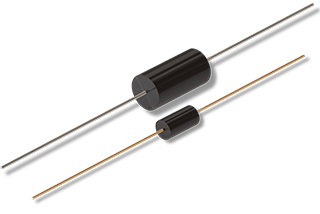Precision Resistor ASTRO2 - wirewound
Wired resistor with extreme wide resistance range of 0.01 Ω…6 MΩ, tolerance up to ±0.005% and max. working voltage of 900V
• Resistance range of 0.01 Ω...6 MΩ
• Exact values up to 0.005% resistance tolerance
• Temperature coefficient up to ±10ppm/°C
• Very long-term stable ±100ppm/year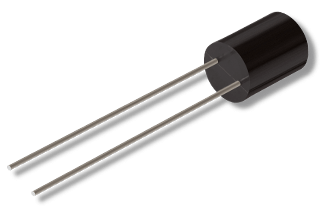Precision Resistor ASTRO5 - wirewound
Radial-wired resistor with wide resistance range of 0.01 Ω…1 MΩ and tolerance up to ±0.005%
• Resistance range of 0.01 Ω...1 MΩ
• Exact values up to 0.005% resistance tolerance
• Temperature coefficient up to ±10ppm/°C
• Very long-term stable ±100ppm/year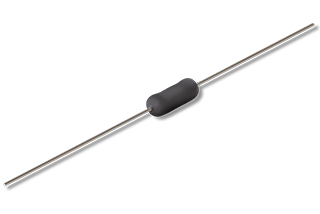Power Resistor SUT - wirewound
Compact 15 watt power resistor with 0.01 Ω…260 kΩ, max. -55 °C…+250 °C, max. working voltage 1050V - does not require a heat-sink
• Power resistor with up to 15 watts
• Space-saving design
• Very good impulse behaviour
• Standard tolerance ±0.01%…±10%
• Temperature coefficient from ±20ppm/°C
• Non-inductive windings (option)
• Resistance values of 0.01 Ω…260 kΩ
• Max. -55 °C…+250 °C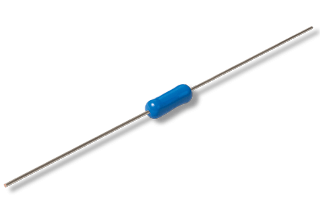Precision Resistor NC550 - metal film
Metal film resistor with wide resistance range of 1 Ω…5 MΩ and resistance tolerance up to ±0.05%
• Resistance values of 1 Ω…5 MΩ
• Resistance tolerances up to ±0,05%
• Temperature coefficient from ±3ppm/°C
• Rated load 0.6 watts @40 °C
• Low inductance version (optional)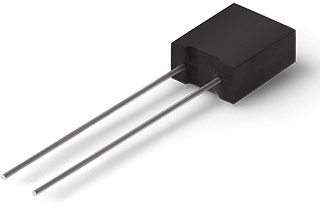Precision Resistor UMX - metal film
Radial wired resistor with resistance range of 10 Ω…1 MΩ, resistance tolerance up to ±0,01% and max.working voltage of 500V
• Resistance values of 10 Ω…1 MΩ
• Temperature coefficient from ±5ppm/°C
• Power rating up to 0.5 Watt @70 °C
• Low induction and capacitive free design
• Robust epoxy housingPower Resistor UT - wirewound
10 watt high temperature power resistor for max. -55 °C…+350 °C with wide resistance range 0.01 Ω…260 kΩ - does not require a heat-sink
• Power resistor with up to 10 watts
• Very good impulse handling
• Resistance tolerance ±0,01%…10%
• Temperature coefficient ±20ppm/°C
• Resistance values of 0.01 Ω…260 kΩ
• Max. working voltage of 850V
• Non-inductive windings (option)
• Option (HT) temperature range from -55 °C…350 °C
• Option 4-pin (Kelvin) connection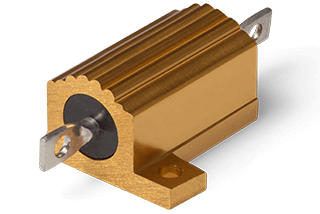Power Resistor MAL - wirewound
50 watt power resistor in aluminium housing with very high temperature range of -55 °C…+275 °C
• Power up to 50 watts with heat-sink
• Resistance range from 0.01 Ω up to 250 kΩ
• Resistance tolerance from ±0.01%
• Standard TC from ±20ppm/°C
• Sturdy aluminium housing
• Low inductive winding (option)
• Numerous connection variants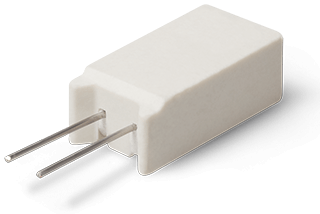Power Resistor MCV - wirewound
10 watt @75 °C power resistor in flame-proofed ceramic housing with very high temperature range -55 °C…+275 °C - does not require a heat-sink!
• Flame-proofed - wirewound resistor cemented in ceramic
• Power rating up to 10 watts @75 °C
• Does not need a heat sink
• Resistance values of 0.01 Ω…90 kΩ
• Resistance tolerance up to ±0.01%
• Temperature coefficient ±20ppm/°C
• Non-inductive windings (option)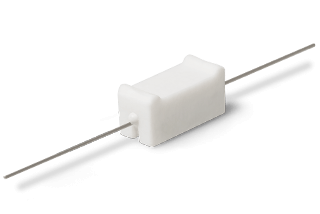Power Resistor MCU - wirewound
15 watt @75 °C power resistor in flame-proofed ceramic housing with very high temperature range -55 °C…+275 °C - does not require a heat-sink!
• Flame-proofed - wirewound resistor cemented in ceramic
• Power rating up to 15 watts @75 °C
• Does not need a heat sink
• Resistance values of 0,01 Ω…90 kΩ
• Resistance tolerance up to ±0,01%
• Temperature coefficient ±20ppm/°C
• Non-inductive windings (option)
• With spacer (option)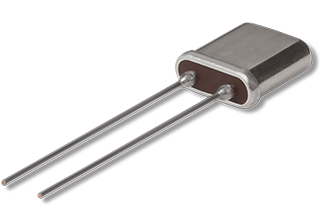Ultra Precision Resistor MZH - metal foil
Ultra-precise zero-TCR-resistor with resistance tolerance up to ±0.005% in metal housing
• TCR of almost zero
• Metal foil resistor with high long-term stability
• Resistance range 100 Ω…100 kΩ
• High precision, resistance tolerances from ± 0.005%
• Hermetically sealed metal housing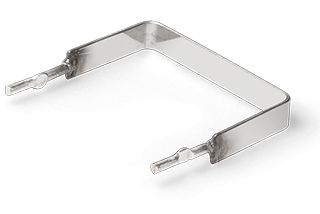Shunt Resistor MST - bare metal
Bare metal shunt resistor with power rating up to 5 watts @85 °C, tolerances of ±1%…±5% for resistance values of 0.005 Ω…0.1 Ω
• Bare metal resistor
• Current sense resistor
• Power rating up to 5 watts @85 °C
• Resistance values of 0.005 Ω…0.1 Ω
• Resistance tolerance of ±1%, ±5%
• Low inductance <10nH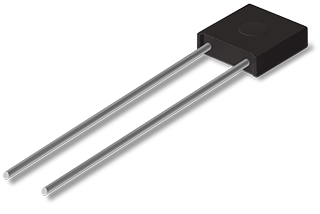Precision Resistor MR - metal foil
Metal foil resistor with extended temperature range of -65 °C…+175 °C, power rating up to 0,5W @125 °C and wide TC range of ±1ppm…±15ppm
• Temperature coefficient up to ±1ppm/°C
• Very high long-term stability
• Resistance values from 1 Ω…400 kΩ
• Exact values up to ±0,005% resistance tolerance
• Low current noise
• Robust epoxy housing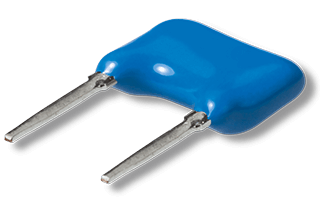Precision Resistor MFL - metal foil
Metal foil resistor with low TC value up to ±2.5ppm/°C and resistance range of 10 Ω…200 kΩ
• Very high long-term stability
• Resistance values of 10 Ω…200 kΩ
• Exact values up to ±0,05% resistance tolerance
• Temperature coefficients from ±2,5ppm/°C
• Low current noise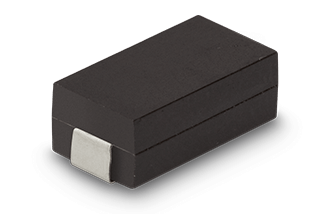SMD Resistor MSI - wirewound
Flame-proofed SMD resistor with load capacity up to 4W @70 °C, very small resistance values from 0.005 Ω, very high temperature range -55 °C…+275 °C
• With power up  to 4 watts
• Increased working temperature range -55 °C…+275 °C
• Flame-proofed UL94 V-0
• Resistance values from 0.005 Ω…50 kΩ
• Non-inductive windings (option)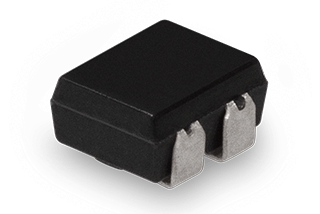SMD resistor MMU as voltage divider - metal foil
Very temperature-stable voltage divider up to 0,05W @125 °C in SMD design excellent under difficult environmental conditions
• SMD precision voltage divider
• Resistance values of 10 Ω…20 kΩ
• Temperature coefficient from ±5ppm/°C absolute, matching ±1ppm/°C
• Robust epoxy housing
• Low induction and low noise
• Very high accuracy and stability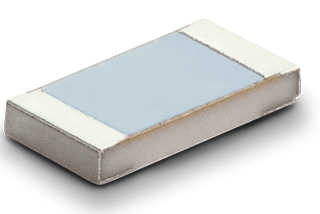SMD Resistor CPK - metal foil
SMD metal foil resistor with excellent long term stability in 0603, 0805 or 1206 design with exactly definable values e.g. 3k529Ω
• High precision metal foil resistor
• Resistance tolerance up ±0,01%
• TCR rate up to ±2ppm/°C
• Excellent long term stability ∆R ±0,005% @2000 h rated power
• Exactly definable values e.g. 3k529Ω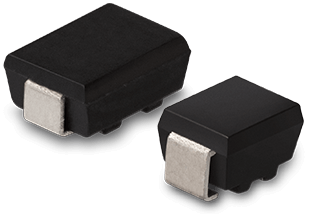SMD Resistor MMP/MMQ - metal foil
Very temperature stable SMD resistor up to +125 °C and increased working temperature range of -65 °C…+175 °C with free bent terminals
• Very good long-term stability
• Free bent terminals
• Resistance values from 30 Ω…60 kΩ
• Resistance tolerance from ±0,05%
• Temperature range -65 °C…+175 °C
• ≥ ±1ppm/°C optional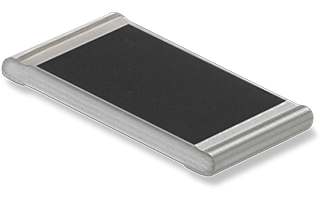SMD Resistor CPH - thin film
SMD resistor with excellent long-term stability, up to ±1ppm/°C and max. working voltage of 300V in sizes 0603, 0805, 1206, 2010
• Ultra-precise NiCr chip resistor
• Low noise construction
• Resistance tolerance up to ±0.01%
• Temperature coefficient up to ±1ppm/°C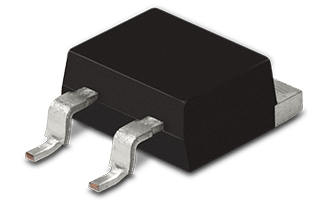Power Resistor M35 - metal film
35 watt power resistor @25 °C in TO-263 housing and max. working voltage of 500V
• High power, up to 35 watts (on heat-sink)
• Resistance range 0.01 Ω…51 kΩ
• Resistance tolerance from ±1%
• Temperature coefficient from ±50ppm/°C
• TO-263 housing (D-PAK)
• Low inductance (<10nH)
• Solder resistance in reflow process at 260 °C / 20 sec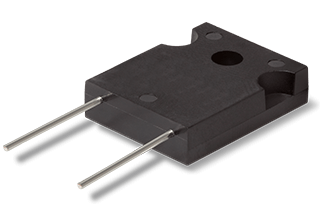Power Resistor M247 - metal film
140 watt power resistor @25 °C in TO-247 housing and max. working voltage of 700V
• Power rating up to 140 watts (on heat-sink)
• Resistance range 0.02 Ω...51 kΩ
• Resistance tolerance from ±1%
• Temperature coefficient from ±50ppm/°C
• TO-247 housing
• Low inductance (<50nH)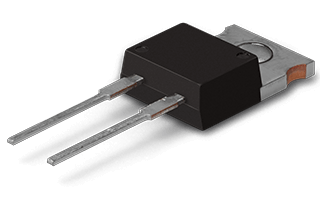Power Resistor M220 - metal film
50 watt power resistor @25 °C in TO-220 housing and max. working voltage of 500V
• Power rating up to 50 watts (on heat-sink)
• Resistance range 0.01 Ω…51 kΩ
• Resistance tolerance from ±1%
• Temperature coefficient from ±50ppm/°C
• TO-220 housing
• Low inductance (<10nH)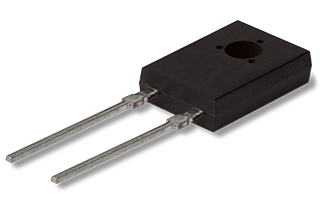Power Resistor M126 - metal film
20 watt power resistor @25 °C in TO-126 housing and max. working voltage of 500V in compact design
• Power rating up to 20 watts (on heat-sink)
• Small dimensions and flat design
• Resistance range 0.01 Ω...51 kΩ
• Resistance tolerance from ±1%
• Temperature coefficient from ±50ppm/°C
• TO-126 housing
• Low inductance (<50nH)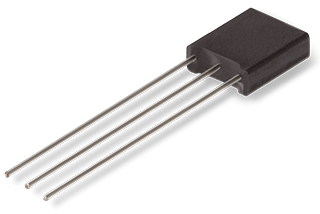Voltage Divider MSM - metal foil Resistor
Very temperature-stable 2-fold voltage divider up to +125 °C with max. 30 kΩ resistance in moisture-resistant epoxy housing
• High load capacity up to +125 °C
• Resistance values of 50 Ω…30 kΩ
• TCR from ±2.5 ppm/°C absolute
• Resistance tolerance absolute from ±0.02%, matching from ±0.01%
• Low noise with good long-term stability
• Solid and moisture resistant epoxy housing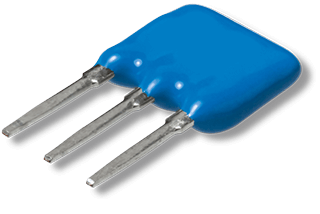Voltage Divider MLD - metal foil Resistor
2-fold voltage divider with max. 30 kΩ resistance value
• 2-fold voltage divider
• Resistance values of 50 Ω…30 kΩ
• Absolute TCR from ±2.5ppm/°C
• Resistance tolerance absolute from ±0,05%, matching from ±0,02%
• Low noise with good long-term stability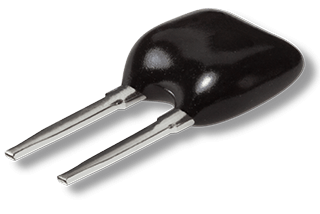Thermistor PTC resistor TM - metal foil
Ultra precision and linear thermistor PTC resistor with TCR up to +4250…+6590ppm/°C
• Thermistor with high long-term stability
• High precision, resistance tolerances from ±0,5% @0 °C
• Temperature coefficient up to 6590ppm @0°…100 °C
• Linear temperature behaviour
• Short response time
• Customized temperature characteristics (on request)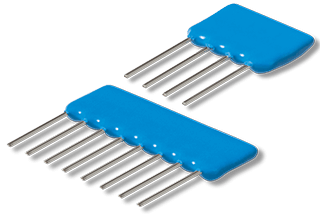Resistor Network MONO - metal film
Thin film networks in monolithic architecture, particularly low-noise and very stable components
• In small quantities available
• Customized circuit layout
• Any combination of resistance values
• Short delivery times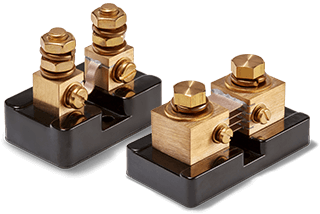Shunt Resistor SMB
Shunt resistor with rated current with up to 1200 A for rated output 50 or 100 mV @ ±0.25% voltage tolerance
• DC Ammeter Shunts
• Manganin Resistive Element
• Non-Inductive Metal Element
• Rated current up to 1200 A
• Rated Output 50mV, 100mV or customized
• Voltage tolerance ±0.25% (optional ±0.1%)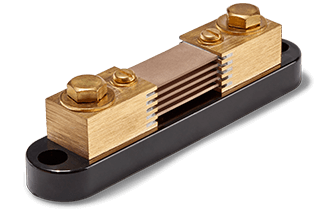Shunt Resistor SMA
Shunt resistor with rated current with up to 500 A for rated output 50 or 100 mV @ ±0.1% voltage tolerance
• DC Ammeter Shunts
• Manganin Resistive Element
• Non-Inductive Metal Element
• Rated Output 50mV, 100mV or customized
• Voltage tolerance ±0.1%
My products
Close